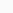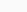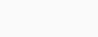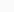# What is the room equivalent absorption area?

## Definition

The room equivalent absorption area,, is used to characterize the acoustic absorption capacity of a room. The acoustic absorption of a given room is linked to its wall covering, its size, the furniture, etc. The equivalent absorption area corresponds to the area in m2 of an imaginary surface with an absorption coefficient of 1 which would provide the same overall absorption as the actual room absorption.

In practice, the absorption coefficient is obtained from reverberation time () measurements inside the room, by using the Sabine’s formula:whereis the volume of the room, in m3.

## Application to e-NVH

Equivalent absorption area is required to convert Sound Power Level into Sound Pressure Level in a room with significant reverberation.

## Application to MANATEE

Manatee software allows calculating both sound power and sound pressure levels.

What is the difference between Sound Pressure Level (SPL) and Sound Power Level (SWL)?